Courses

# Light : Reflection And Refraction - 1

## 10 Questions MCQ Test Science Class 10 | Light : Reflection And Refraction - 1

Description
This mock test of Light : Reflection And Refraction - 1 for Class 10 helps you for every Class 10 entrance exam. This contains 10 Multiple Choice Questions for Class 10 Light : Reflection And Refraction - 1 (mcq) to study with solutions a complete question bank. The solved questions answers in this Light : Reflection And Refraction - 1 quiz give you a good mix of easy questions and tough questions. Class 10 students definitely take this Light : Reflection And Refraction - 1 exercise for a better result in the exam. You can find other Light : Reflection And Refraction - 1 extra questions, long questions & short questions for Class 10 on EduRev as well by searching above.
QUESTION: 1

### A concave mirror is used to form an image of the sun on a white screen. If the lower half of the mirror is covered with a black paper, the effect on the image formed on the screen would be:

Solution:

If the lower half (or a part) of a mirror is covered with a black paper then a complete image of the object will be formed as before. However, the image will be less bright now.

QUESTION: 2

### Figure shows a ray of light as it travels from medium A to medium B. Refractive index of the medium B relative to medium A is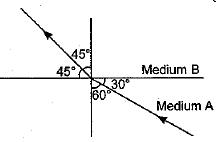Solution:

Here, ∠i = 60°, ∠r = 45°
Using Snell’s law of refraction, refractive index of medium B with respect to medium A.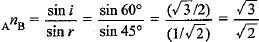QUESTION: 3

### A full length image of a distant tall building can definitely be seen by using:

Solution:

A full length image of a distant tall building can definitely be seen by using a convex mirror because a convex mirror always forms a virtual, erect and highly diminished image.

QUESTION: 4

The angle of incidence of any light ray passing through the centre of curvature of a spherical mirror is:

Solution:

The angle of incidence of any light ray passing through the centre of curvature of a spherical mirror is 0°, because a line joining centre of curvature to any point on the mirror is a normal drawn at that point of the mirror.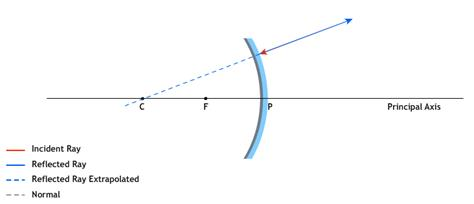QUESTION: 5

A 10 mm long awl pin is placed vertically in front of a concave mirror. A 5 mm long image of the awl pin is formed at 30 cm in front of the mirror. The focal length of this mirror is:

Solution:

Given h0 = +10 mm - + 0.1 cm
h2 = - 5 mm = -0 .5 cm
for real image, v = - 30 cm

Now magnification,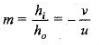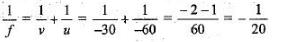∴ f = -20 cm

QUESTION: 6

In case of a real and inverted image the magnification of a mirror is:

Solution:

Magnification is negative for a real and inverted image formed by a mirror.

QUESTION: 7

The distance between the optical centre and point of convergence is called focal length in which of the following cases?

Solution:

Parallel rays after refracting meet at the focus of a convex lens.

QUESTION: 8

Rays from sun converge at a point 15 cm in front of a concave mirror. Where should an object be placed so that size of the image is exactly equal to the size of the object ?

Solution:

As light rays from sun (u = ) converge at a point 15 cm in front of a concave mirror, hence focal length of concave mirror f = - 15 cm.
To form an image of exactly same size as that of an object, the object should be placed at the centre of curvature (u = R = 2f) of mirror. Hence, the object should be placed at 30 cm in front of mirror.

QUESTION: 9

A magnified real image is formed by a convex lens when the obiect is at:

Solution:

To get the real and magnified image for a convex lens, the object is placed in between F and 2F.

QUESTION: 10

A virtual, erect and magnified image of an object is to be produced with a concave mirror of focal length 12 cm. Object may be placed at a distance of:

Solution:

A concave mirror forms a virtual, erect and magnified image when an object is placed between pole and focus point of the mirror. As focal length of given concave mirror, hence object must be placed at a distance less than 12 cm i.e., u < 12 cm. Thus, the object may be placed at a distance of 10 cm from the mirror.